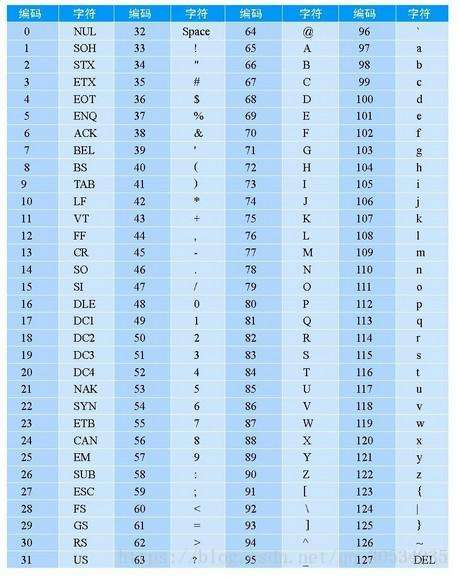## 一、string转char*

3.1 data()方法

``````string str = "hello";
//使用char * p=(char*)str.data()效果相同const char* p = str.data();123
``````

3.2 c_str()方法

``````string str=“world”;//使用char * p=(char*)str.c_str()效果相同const char *p = str.c_str();123
``````

3.3 copy()方法

``````string str="world";char p;//s.copy(cstr, n, pos)       从字符数组cstr的pos位置开始，复制n个字符到字符串s中str.copy(p, 5, 0);
*(p+5)=‘\0’;                 //添加结束符12345
``````

## 二、char * 转string

``````string s;char *p = "helloworld";
s = p;123
``````

## 三、string转char[]

for循环遍历输入。

``````string pp = "helloworld";char p;int i;for( i=0;i<pp.length();i++)
p[i] = pp[i];
p[i] = '\0';                  //添加结束符123456
``````

## 四、char[]转string

``````string s;char p = "helloworld";
s = p;123
``````

## 五、char[]转char*

``````char pp = "helloworld";char* p = pp;12
``````

## 六、char*转char[]

6.1 strcpy()方法

``````char arr;char* tmp = "helloworld";strcpy(arr, tmp);123
``````

6.2 循环遍历

``````char arr;char* tmp = "helloworld";int i = 0;while (*tmp != '\0')
arr[i++] = *tmp++;
arr[i] = '\0';             //添加结束符
``````

## 七、char转int

char转int之前，先将运算式中的每个字符都转换成ASCII码值，再进行计算。 以下代码为例，其中i3的结果符合我们的预期要求。

``````char c = '0';

int i1 = c;                    // 48
int i2 = c - 0;                // 48
int i3 = c - '0';              // 0
int i4 = c + '0';              // 96123456
``````

## 八、int转char

int转char之前，先将运算式中的每个字符都转换成ASCII码值，再进行计算。 计算出数值后，再据此转换为字符（数值为该字符对应的ASCII码值）。 以下代码为例，其中c3的结果符合我们的预期要求。

``````int i = 5;

char c1 = i;                  // 越界
char c2 = i - 0;              // 越界
char c3 = i - '0';            // 越界
char c4 = i + '0';            // 5
``````

## 九、字符串拼接

``````string str1, str2, str3;
str1 = str2 + str3;    // 加法拼接
str1 += str2;          // 加法拼接
``````

## 附、ASCII 码表